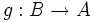# Homotopy equivalence of topological spaces

## Definition

Suppose$A$ and$B$ are topological spaces. A homotopy equivalence between$A$ and$B$ is a map$f:A \to B$ such that there exists a map$g:B \to A$ for which$f \circ g$ is homotopic to the identity on$B$ and$g \circ f$ is homotopic to the identity on$B$.

Two topological spaces between which there exists a homotopy equivalence are termed homotopy-equivalent topological spaces.